# Order-4 hexagonal tiling

Order-4 hexagonal tiling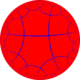Poincaré disk model of the hyperbolic plane
TypeHyperbolic regular tiling
Vertex figure64
Schläfli symbol{6,4}
Wythoff symbol4 | 6 2
Coxeter diagramSymmetry group[6,4], (*642)
DualOrder-6 square tiling
PropertiesVertex-transitive, edge-transitive, face-transitive

In geometry, the order-4 hexagonal tiling is a regular tiling of the hyperbolic plane. It has Schläfli symbol of {6,4}.

## Symmetry

This tiling represents a hyperbolic kaleidoscope of 6 mirrors defining a regular hexagon fundamental domain. This symmetry by orbifold notation is called *222222 with 6 order-2 mirror intersections. In Coxeter notation can be represented as [6*,4], removing two of three mirrors (passing through the hexagon center). Adding a bisecting mirror through 2 vertices of a hexagonal fundamental domain defines a trapezohedral *3322 symmetry. Adding 3 bisecting mirrors through the vertices defines *443 symmetry. Adding 3 bisecting mirrors through the edge defines *3222 symmetry. Adding all 6 bisectors leads to full *642 symmetry.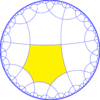*222222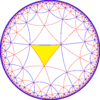*443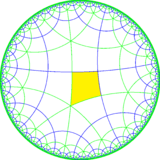*3222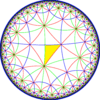*642

## Uniform colorings

There are 7 distinct uniform colorings for the order-4 hexagonal tiling. They are similar to 7 of the uniform colorings of the square tiling, but exclude 2 cases with order-2 gyrational symmetry. Four of them have reflective constructions and Coxeter diagrams while three of them are undercolorings.

Uniform constructions of 6.6.6.6
1 color 2 colors 3 and 2 colors 4, 3 and 2 colors
Uniform
Coloring(1111)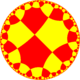(1212)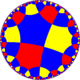(1213)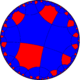(1113)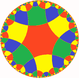(1234)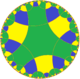(1123)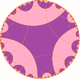(1122)
Symmetry [6,4]
(*642)[6,6]
(*662)=[(6,6,3)] = [6,6,1+]
(*663)=[1+,6,6,1+]
(*3333)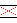==Symbol {6,4} r{6,6} = {6,4}1/2 r(6,3,6) = r{6,6}1/2 r{6,6}1/4
Coxeter
diagram=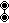===This tiling is topologically related as a part of sequence of regular tilings with hexagonal faces, starting with the hexagonal tiling, with Schläfli symbol {6,n}, and Coxeter diagram, progressing to infinity.

This tiling is also topologically related as a part of sequence of regular polyhedra and tilings with four faces per vertex, starting with the octahedron, with Schläfli symbol {n,4}, and Coxeter diagram, with n progressing to infinity.Wikimedia Commons has media related to Order-4 hexagonal tiling.